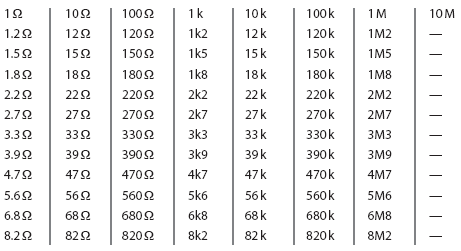# ResistorsElectronics guide > The very first steps > Resistors

The components which are used as resistances are called, naturally enough, resistors. So that we can control current and voltage in specified ways, resistors are available in a number of values. Obviously, it would be impractical to have resistors of every possible value (for example, 1 Ω , 2 Ω , 3 Ω, 4 Ω) because literally hundreds of thousands — if not millions — of values would have to exist.

Instead, agreed ranges of values exist: and manufacturers make their resistors to have those values, within a certain tolerance. Table 1.3 (over) shows a typical range of resistor values, for example. This range is the most common. You can see from it that large values of resistors are available, measured in kilohms, that is, thousands of ohms (unit: kΩ) and even megohms, that is, millions of ohms (unit: MΩ). Sometimes the unit Ω (or the letter R if used) is omitted, leaving the units as just k or M.

Resistor tolerance is specified as a plus or minus percentage. A 10 Ω ±10% resistor, say, may have an actual resistance within the range 10 Ω –10% to 10 Ω +10%, that is, between 9 Ω and 11 Ω .

As well as being rated in value and tolerance, resistors are also rated by the amount of power they can safely dissipate as heat, without being damaged. As you’ll remember from our discussion on soldering irons earlier, power rating is expressed in watts (unit: W), and this is true of resistor power ratings, too.Table 1.3 Typical resistor value range

As the currents and voltages we use in electronics are normally pretty small, the resistors we use also have small power ratings. Typical everyday resistors have ratings of 1/4 W, 1/3 W, 1/2 W, 1 W and so on. At the other end of the scale, for use in power electrical work, resistors are available with power ratings up to and over 100 W or so.

Choice of resistor power rating you need depends on the resistor’s use, but a reasonable value for electronics use is 1/4 W. In fact, 1/4 W is such a common power rating for a resistor that you can assume it for all the circuits in this book. If I give you a circuit to build which uses resistors of different power ratings, I’ll tell you.

<< Electronic components